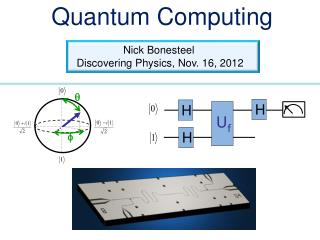DownloadDownload PresentationQuantum Computing

# Quantum Computing

Download Presentation## Quantum Computing

- - - - - - - - - - - - - - - - - - - - - - - - - - - E N D - - - - - - - - - - - - - - - - - - - - - - - - - - -
##### Presentation Transcript

1. Quantum Computing Uf Nick Bonesteel H H Discovering Physics, Nov. 16, 2012 q H f

2. What is a quantum computer, and what can we do with one?

3. Single Bit Operation: NOT NOT x y 0 1 1 0 y x 0

4. Single Bit Operation: NOT NOT x y 0 1 1 0 y x 1

5. A Quantum Bit or “Qubit” Quantum superposition of 0 and 1 0 1

6. A Quantum Bit: A Continuum of States q 0 1 cos sin q q +

7. q q - f + i cos 0 sin e 1 2 2 A Quantum Bit: A Continuum of States Actually, qubit states live on the surface of a sphere. q f

8. A Quantum Bit: A Continuum of States But the circle is enough for us today. q 0 1 cos sin q q +

9. X A Quantum NOT Gate

10. X X A Quantum NOT Gate

11. X A Quantum NOT Gate

12. X X A Quantum NOT Gate

17. H H Hadamard Gate H is its own inverse

18. H H Hadamard Gate H is its own inverse

19. H H Hadamard Gate H is its own inverse

20. H H Hadamard Gate H is its own inverse

21. Fair Coin Trick Coin

22. Balanced Function or Unbalanced Function or

23. Uf A Two QubitSubroutine to Evaluate f(x)

24. Uf A Two QubitSubroutine to Evaluate f(x) Input x can be either 0 or 1 Output is f(x) Initialize to state “0”

25. Uf A Two QubitSubroutine to Evaluate f(x) Input x can be either 0 or 1 This qubit can also be in state “1”

26. Uf A Two QubitSubroutine to Evaluate f(x) Input x can be either 0 or 1 Bar stands for “NOT” This qubit can also be in state “1” 0 = 1, 1 = 0

27. Uf Uf A Two QubitSubroutine to Evaluate f(x) Unbalanced Balanced or or

28. Uf A Quantum Algorithm (Deutsch-Jozsa ‘92) H H H

29. Uf A Quantum Algorithm (Deutsch-Jozsa ‘92) H H H

30. Uf A Quantum Algorithm (Deutsch-Jozsa ‘92) H H H

31. Uf A Quantum Algorithm (Deutsch-Jozsa ‘92) H H H

32. Uf A Quantum Algorithm (Deutsch-Jozsa ‘92) H H H

33. Uf A Quantum Algorithm (Deutsch-Jozsa ‘92) H H H Only ran Uf subroutine once, but f(0) and f(1) both appear in the state of the computer!

34. Uf A Quantum Algorithm (Deutsch-Jozsa ‘92) H H H If f is balanced: f(0) = f(1) and f(0) = f(1)

35. Uf A Quantum Algorithm (Deutsch-Jozsa ‘92) H H H If f is balanced: f(0) = f(1) and f(0) = f(1)

36. Uf A Quantum Algorithm (Deutsch-Jozsa ‘92) H H H If f is balanced: f(0) = f(1) and f(0) = f(1)

37. Uf A Quantum Algorithm (Deutsch-Jozsa ‘92) H H H If f is unbalanced: f(0) = f(1) and f(0) = f(1)

38. Uf A Quantum Algorithm (Deutsch-Jozsa ‘92) H H H If f is unbalanced: f(0) = f(1) and f(0) = f(1)

39. Uf A Quantum Algorithm (Deutsch-Jozsa ‘92) H H H If f is unbalanced: f(0) = f(1) and f(0) = f(1)

40. Uf A Quantum Algorithm (Deutsch-Jozsa ‘92) H H H Balanced: Unbalanced:

41. Uf A Quantum Algorithm (Deutsch-Jozsa ‘92) H H H Balanced: Unbalanced: